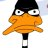# I need a math genius!

#### Da Foxx

##### Well-Known Member
OP
Member
Alright I didn't do my homework because I was working Saturday and Sunday and I just got off work. I have no idea what I'm doing. I was hoping someone can help me out... I'm trying to do it now but its completely wrong.

Pic 1
Pic 2

4 questions in total...

#### FAST6191

##### Techromancer
Editorial Team
I dislike doing other peoples work but I have no objection to helping out. SO no full answers but you should be able to get them easily enough.

Pic1 first question.
Unless you are dividing you can usually consider things involving greater/less than as normal equations (diving just occasionally means you have to flip signs) and then stipulate once all is said and done.

The vertical lines are modulus lines (i.e. make postive). You might know it as absolute however and a lot of computers do not take modulus (mod usually means mode) so I would learn both.

|4a+2| - |a-2| > 1

Three things to account for.
Duality from modulus i.e. 7=|-7|, the inequality and the fact you have two terms using the same variable.
Assume a basic equation now as we can sort the inequality later.
I am being a bit lazy today so go through the example on http://www.purplemath.com/modules/solveabs2.htm (the first part of the page you can ignore, the second example is what you want. Read the previous page too if you still are a bit hazy on it)
Once all is done from there account for the inequality and you are done.

You might find it easier to first change your equation to match that example.
second question

(2x-3)^1/2 + (x+2)^1/2 = 5

Restrictions I assume come in to play as you are probably not going to be allowed imaginary/complex numbers. I.e. none of the bracketed terms can solve to be negative: 2x-3>0 and x+2>0 aka x>3/2

From here is is basically a ye olde algebra solving, be especially careful signs though as this is not a nice one.
I stuck root x+2 on the other side. Squared it.
2x-3 = 25 - 10(x+2)^1/2 + x + 2
rinse and repeat until you get a quadratic and insert into usual quadratic equation/quadratic formula x=-b........

One of my answers was just over 4.5 and I got rid of the other by mistake.

Question 3
Bear in mind p can not equal 2 or you end up with a divide by zero.
algebra now. If you like this is basic fraction maths.
Only way to get 0 with a fraction is to have the numerator equalling zero (assuming no infinity).
Back again to quadratics.
p^2-3p-10=0
a=1, b=-3 c=-10
answers, -2 and 5.
So you now have the turning points and can do what you like with them.

4. I am lazy right now so spreadsheet it is. Again I assume imaginary/complex numbers are not an option.
Therefore the graph does not exist below x=0.5
By and large graph questions are all about the graph so make sure to label axes, title it and whatnot.Asymptote definition: http://mathworld.wolfram.com/Asymptote.html and http://www.freemathhelp.com/asymptotes.html
Rearrange the equation give to get 2x-1=16 and solve for the x intercept, there is no y intercept.

Replies
1
Views
114
Replies
0
Views
162
Replies
8
Views
791
Replies
2
Views
812
Replies
8
Views
2K

### Recent Content

12. #### wbfs2fat.py

Help Users
• No one is chatting at the moment.@ KenniesNewName: Well new hocus pocus already looks like shit